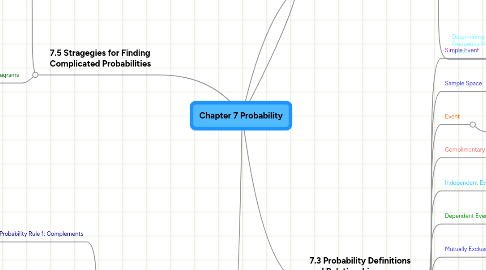# Chapter 7 ProbabilityTuong Vi Nguyen
Get Started. It's FreeChapter 7 Probability## 1. 7.1 Random Circumstances

### 1.1. Random Circumstance

1.1.1. When the outcome is unpredictable, the outcome is not determined until we observe it

### 1.2. Probability

1.2.1. How likely it is that a particular outcome will be the result of a random circumstance. The total of the assigned probablities must equal 1

## 2. 7.5 Stragegies for Finding Complicated Probabilities

### 2.1. Bayes Rule

2.1.1. P(A given B) = P(A and B)/[P(B given A)*P(A) + P(B given A^c)*P(A)}

### 2.2. Tree Diagrams

2.2.1. A schematic representation of the sequence of events and their probabilities, including conditional probabilities based on previous events for the events that happen sequentially

2.2.2. Steps for creating a tree diagram

2.2.2.1. Determine the first random circumstance in the sequence, and create the first set of branches to illustrate possible outcomes for it. Create one branch for each outcome, and write the associated probability on the branch

2.2.2.2. Determine the next random circumstance, and append the branches for the possible outcomes to each of the branches in step 1.

2.2.2.3. Continue this process for as many steps as necassary

2.2.2.4. To determine the probability of any particular sequence of branches, multiply the probabilities on those branches

2.2.2.5. To determine the probability of any collection of sequences of branches, add the individual probabilities for those sequences

## 3. 7.4 Basic Rules for Finding Probabilities

### 3.1. Probability Rule 1: Complements

3.1.1. (for "not the event") To find the probability of A^c, the complement of A, use P(A^C) = 1 - P(A). P(A^c) is the probability that the event will not occur

### 3.2. Probability Rule 2: Addition

3.2.1. Rule 2a

3.2.1.1. (General) P(A+B) = P(A) + P(B) - P(A and B)

3.2.2. Rule 2b

3.2.2.1. (Mutually Exclusive Events) If A and B are mutually exclusive events, P(A or B) = P(A) + P(B)

### 3.3. Probability Rule 3: Multiplication

3.3.1. To find the probability that two events A and B both occur simultaneously or in a sequence

3.3.2. Rule 3a

3.3.2.1. P(A and B) = P(A)*P(B given A) or P(A and B) = P(B)*P(A given B)

3.3.3. Rule 3b

3.3.3.1. For INDEPENDENT events

3.3.3.2. P(A and B) = P(A)*P(B)

### 3.4. Probability Rule 4: Conditional Probability

3.4.1. P(B given A) = P(A and B)/P(A) or P(A given B) = P(B and A)/P(B)

### 3.5. Sample without Replacement

3.5.1. When individuals are not eligible for subsequent selection

### 3.6. Sample with Replacement

3.6.1. When individuals are returned to the eligible pool for each selection

## 4. 7.2 Interptetations of Probability

### 4.1. Relative Frequency

4.1.1. For situations that we can imagine repeating many times, we define the probability of a specific outcome as the proportion of times it would occur over the long run (numbers between 0 and 1)

### 4.2. Personal Probability

4.2.1. The degree to which a given individual believes that the event will happen

### 4.3. Subjective Probability

4.3.1. Another term used for personal probability because the degree of belief may be different for each individual

### 4.4. Coherent Probabilities

4.4.1.1. EXAMPLE If you believe the probability of getting an A in Statistics is .4 then you must also believe the probability of not getting an A is .6 if they are to be coherent probabilities.

### 4.5. Determining the Relative Frequency Probability of an outcome

4.5.1. Making an assumption about the physical world

4.5.1.1. EXAMPLE The probability of a flipped coin showing heads up is 1/2

4.5.2. Making a direct observation of how ofter something happens

4.5.2.1. EXAMPLE (according to the book) The probability that a live birth will result in a male is about .5117

## 5. 7.3 Probability Definitions and Relationships

### 5.1. Simple Event

5.1.1. One outcome in the sample space, a possible outcome of a random circumstance

### 5.2. Sample Space

5.2.1. The collection of unique overlapping possible outcomes of a random circumstance

### 5.3. Event

5.3.1. A collection of one or more simple events in the sample space(Events are often written using capital letters A,B,C, & ect.)

### 5.4. Complimentary events

5.4.1. Opposite events EXAMPLE you have blonde hair or you don't have blonde hair

### 5.5. Independent Events

5.5.1. If knowing that one will occur or has occurred does not change the probability that other will occur

### 5.6. Dependent Events

5.6.1. If knowing that one will occur or has occurred changes the probability that the other occurs

### 5.7. Mutually Exclusive (Disjoint) Events

5.7.1. Do not contain any of the same simple events (outcomes)

### 5.8. Complement

5.8.1. When two events do not contain any of the same simple events and together they cover the entire sample space (For an event A, the notation A^c represents the complement of A)

5.8.1.1. Probabilities of Compliment events must sum to 1. P(A) + P(A^c) = 1 so P(A^c) = 1 - P(A)

### 5.9. Assigning probabilities to simple events

5.9.1. P(A) is used to denote the probability that event A occurs

### 5.10. Two conditions for valid probabilities

5.10.1. Each probability is between 0 and 1

5.10.2. The sum of the probabilities over all possible simple events is 1

### 5.11. Conditional Probabilities

5.11.1. P(B), the unconditional probability that the event B occurs

5.11.2. P(BlA), probability of B given A, the conditional probability that the event B occurs given that we know A has occured or will occur

5.11.3. The long-run relative frequency with which event B occurs when circumstances are such that A also occurs